The Diagonal of a Regular Pentagon and the Golden Ratio

We start with a regular pentagon and we want to know the ratio between the diagonal and the side of the pentagon.

We can think that the side of the regular pentagon is 1. And the diagonal is represented by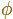, the Greek letter phi.We have two homothetic isosceles triangles: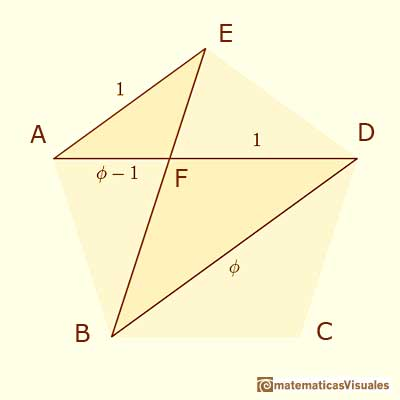We can write a proportion:

Using the numbers 1 and:

Henceis the positive root of the quadratic equation:

The value ofis:

These are some basic properties of:is called the golden ratio or the golden section.

We can say that the diagonal of a regular pentagon are in golden ratio to its sides.

The point of intersection of two diagonals of a regular pentagon are said to divide each other in the golden ratio (or "in extreme and mean ratio").

We suppose that Pythagoras and the Pythagoreans (around 500 BC) knew this ratio because their symbol was the pentagram (a pentagon with its diagonals) but the first written reference we have is in Euclid´s Elements (around 300 BC):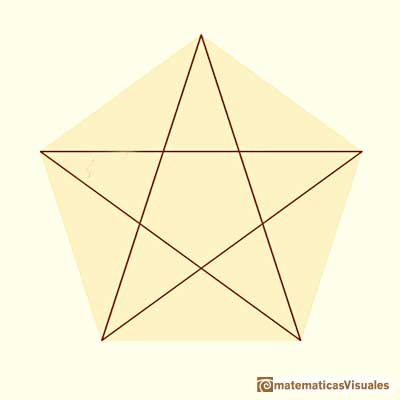Book VI, Definition 3: "A straight line is said to have been cut in extreme and mean ratio when, as the whole line is to the greater segment, so is the greater to the less". (For example, in Euclid's Elements at Clark University by D.E. Joyce)The first reference to this kind of construction appeared in Book II, Proposition 11. That was before ratios were defined and then the proposition is in terms of areas: "To cut a given straight line so that the rectangle contained by the whole and one of the segments equals the square of the remaining segment". (Euclid's Elements at Clark University by D.E. Joyce)

Using our notation:

Usign the golden ratio we can draw a regular pentagon, a golden triangle and a golden rectangle and is related with the icosahedron and the dodecahedron.

With a strip of paper we can make a knot and get a pentagon and a pentagram:Zome is a wonderful tool to play with the golden ratio:Some examples of pentagonal symmetry:REFERENCES

Coxeter - Introduction to Geometry. John Whiley and sons. pp. 160-172.From Euclid's definition of the division of a segment into its extreme and mean ratio we introduce a property of golden rectangles and we deduce the equation and the value of the golden ratio.A golden rectangle is made of an square and another golden rectangle.A golden rectangle is made of an square an another golden rectangle. These rectangles are related through an dilative rotation.The golden spiral is a good approximation of an equiangular spiral.Two equiangular spirals contains all vertices of golden rectangles.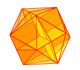The twelve vertices of an icosahedron lie in three golden rectangles. Then we can calculate the volume of an icosahedronSome properties of this platonic solid and how it is related to the golden ratio. Constructing dodecahedra using different techniques.The first drawing of a plane net of a regular dodecahedron was published by Dürer in his book 'Underweysung der Messung' ('Four Books of Measurement'), published in 1525 .He studied transformations of images, for example, faces.Two transformations of an equiangular spiral with the same general efect.In an equiangular spiral the angle between the position vector and the tangent is constant.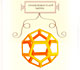Leonardo da Vinci made several drawings of polyhedra for Luca Pacioli's book 'De divina proportione'. Here we can see an adaptation of the dodecahedron.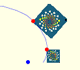A Dilative Rotation is a combination of a rotation an a dilatation from the same point.